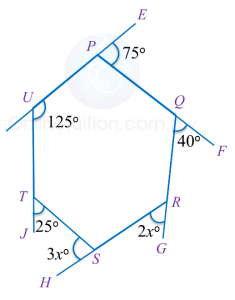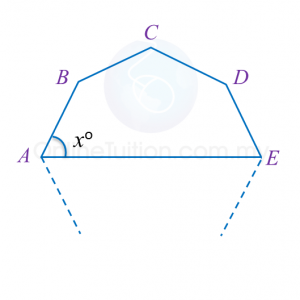2.2.2 Polygons II, PT3 Practice

Question 6:
In diagram below, PQRSTU is a regular hexagon QUV is a straight line.Find the value of x.

Solution:

Question 7:
In diagram below, PQRSTU is a hexagon. UPE, PQF, QRG, RSH and UTJ are straight lines.Find the value of x.

Solution:

Question 8:
In Diagram below, A, B, C, D and E are vertices of a 9 sided regular polygon.Find the value of x.

Solution:

2 thoughts on “2.2.2 Polygons II, PT3 Practice”

1.EASY LAH SOALAN

2.THROW LAH SKIT KBAT